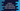# Python program to add separator between parameters while using print() method## How to add a separator between parameters while using print in python:

Python print method provides a way to add one separator between the parameters we are providing to print. It is called sep parameter. This is available in python 3.x and later versions.

If we don’t provide this parameter, it uses a space as the separator.

In this post, we will learn how to use print method and how to add separator with examples.

### Syntax:

Below is the syntax of print:

``print(arg1, arg2,....[,sep])``

Here, we are passing the arguments first before passing the separator. We can pass any number of arguments to the print method.

### Python example program:

Let’s take a look at the below program:

``````print('hello', 'world')

print('hello', 'world', sep='=>')``````

If you run this program, it will print one output as like below:

As you can see here, the first print statement is printing the arguments with space as the separator. But the second print statement is printing the arguments with the separator given.

### Example to print the current hour, minute, seconds with different separators:

Let’s take a look at the below example program:

``````import datetime

now = datetime.datetime.now()
hour = now.hour
minute = now.minute
seconds = now.second

print(hour, minute, seconds)
print(hour, minute, seconds, sep=':')
print(hour, minute, seconds, sep='=>')
print(hour, minute, seconds, sep=',')
print(hour, minute, seconds, sep='---------')``````

Here, we are printing the current hour, minute, and seconds values with different separators.

If you run it, it will print the below output:

``````13 6 51
13:6:51
13=>6=>51
13,6,51
13---------6---------51``````

As you can see, we can add different types of separators to print easily by using the sep parameter.